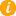# Prime Factorization, Prime Factorization Calculator, Factor Tree## What is Prime Factorization?

Here is a Merriam-Webster Dictionary definition "any of the numbers or symbols in mathematics that when multiplied together form a product". Stated more simply, a large number can be broken down in to a bunch of smaller Prime Numbers; when the smaller numbers are multiplied together, you get the original larger number. Example: the number 12 can be factored into 2 x 2 x 3 = 12. Note 12 is not a prime number but its factors are all prime. This example demonstrates Prime Factorization.

Prime Factorization can be organized in a Factor Tree for ease of viewing. There may be more than one valid factor tree for the same number, but the prime factors at the end are always the same. Before going further, here is recommended reading: Exponents. The factor tree for 108 is shown below:

 108 2 54 2 27 3 9 3 3

As you can see the prime factors of 108 follow: 108 = 3 x 3 x 3 x 2 x 2 = 33 x 22 .

 1 x 108 = 108 2 x 54 = 108 2 x 27 = 54 3 x 9 = 27 3 x 3 = 9

Prime Factorization Calculator
Enter numbers (2 To 1000):
Then Press The SUBMIT Button

Every Natural Number greater than one can be factored in to a product of prime numbers. If the number you are factoring is prime number, you are done. If the number is not prime, factor it and look at each factor. If they are prime, you are done. If they are not prime, repeat the factoring process. Important Factoring Tips:
• if the number being factored ends in 0, factor by 10;
• if the number being factored ends in 5, factor by 5;
• if the number being factored is even, factor by 2 (2 as your factor and the other factor is the number divided by 2 e.g. factors of 6 are 2 and 3);
• if the number being factored is odd, start factoring using 3, if it does not divide evenly, try 4, 5, 6, ... e.g. factors of 21 are 3 and 7.